Next: 5.3.2 Results Up: 5.3 Experiments Previous: 5.3 Experiments

## 5.3.1 Methods

For the tests three synthetic distributions were used, ring-line-square, vortex, and sine-wave, and further data from the kinematic arm model (section 4.5). The ring-line-square distribution is composed of 850 points in a plane. The vortex distribution is also two-dimensional and consists of 700 points. In both sets the points are uniformly distributed in a defined region. The sine-wave is composed of 800 points and is surrounded by 50 outliers (noise). For the kinematic arm model, different from section 4.5, only 5 000 training patterns were generated. Computational limits did not allow a much larger training set (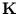is a n×n matrix).

Kernel PCA was done on all data points of each distribution. A Gaussian kernel with width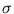was used. The Gaussian kernel corresponds to a mapping into a countable-infinite-dimensional space (section 2.4.3). Thus, according to Cover's theorem (Cover, 1965), the probability to separate linearly (in feature space) the data distribution from its complement is one. This is favorable. The also commonly used polynomial' kernel functions do not have this property (Schölkopf and Smola, 2002). A test with another radial basis function kernel (inverse multi-quadratic', see section 2.4.3), which also fulfills the above property, showed results similar to those gained from the Gaussian kernel. Therefore, the presentation is restricted to the Gaussian function.

The widthwas set to 0.3 for the ring-line-square, the vortex, and the sine-wave distribution (unless otherwise stated) and to 1.5 for the kinematic arm data. The eigenvectors of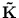were extracted using the Power Method with deflation (appendix B.1).

With the speed-up, the potential is computed from a reduced set of m points {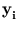} instead of the n data points {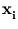} (appendix B.2). To calculate the reduced set, we need to maximize over {}. Schölkopf et al. (1998a) computed {} by iteration. In the present study, however, this was not stable. Sometimes, the iteration ended in an oscillation. Thus, instead, the conjugate gradient method from the Numerical Recipes' code (Press et al., 1993) was used. The values ofwere initialized randomly within the maximum range of the training data. For all tests, the size m of the reduced set was set to n/10.

A quality measure was used to give a quantitative statement on how good a potential field describes the data distribution (appendix B.3). Since we are using uniform distributions, we can define a region that encloses the same volume as the distribution, within an iso-potential curve. The quality is given in percent of the data points covered by that region.

Recall works by solving an optimization problem (see section 5.2.3). The same conjugate gradient method was used to find the parameters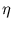*. The components ofwere initially set to zero.

The recall results were compared to the mixture of PCA (chapter 3 and 4). For training, NGPCA was used. Its parameters were, first, for the sine-wave with noise: m = 10 units, q = 2 eigenvectors, tmax = 30 000,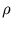(0) = 1.0,(tmax) = 0.001,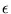(0) = 0.5, and(tmax) = 0.05, and second, for the kinematic arm data: m = 100 units, q = 6 eigenvectors, tmax = 400 000,(0) = 10.0,(tmax) = 0.0001,(0) = 0.5, and(tmax) = 0.001 (the same training parameters as in chapter 4).Next: 5.3.2 Results Up: 5.3 Experiments Previous: 5.3 Experiments
Heiko Hoffmann
2005-03-22